Ben and Jen decided to play a game. They said that they would calculate the product of two facing pages of their textbook and the other would have to guess the page numbers. Ben said the product of two facing pages was 72, and asked Jen to guess the pages he had opened.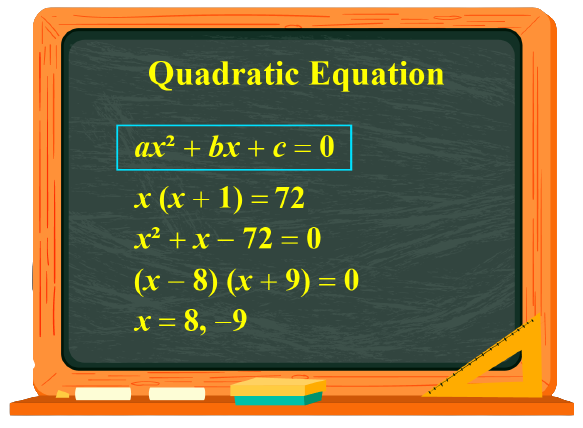Let the page number be $$x$$. Then its next page will be $$x+1$$. Jen observed $$x\times (x+1) =72$$. Then $$x^2 +x =72$$. Thus a quadratic equation is obtained and he has to find the roots by factorizing and get the page numbers to be 8 and 9.

Factorization of a quadratic equation is expressing the equation in terms of linear factors. In this mini-lesson let us learn about the fascinating concept of factorization of quadratic equations. With a lot of examples and the algorithm to be followed, the concept is easier to be explained.

## Lesson Plan

 1 What Is a Quadratic Equation? 2 Important Notes on Quadratic Equations 3 Solved Examples on Quadratic Equations 4 Challenging Questions on Quadratic Equations 5 Interactive Questions on Quadratic Equations

## What Is a Quadratic Equation?

A quadratic polynomial is a polynomial of degree 2.

 $$f(x) = a x^2 + b x + c$$

$$f(x)$$ is the quadratic equation.

### Linear factors

Linear factors are of the form $$ax +b$$ and they cannot be factored further.

They are similar to finding the prime factors of a whole number or an integer.

Linear factors cannot be factored any further.

$$(x+1)$$ is a linear factor of the equation $$x^2+2x+1 = 0$$

Every polynomial can be factored into a product of linear factors and irreducible quadratic factors.

Let us recall how factorization is done with respect to whole numbers, algebraic expressions, and quadratic polynomial.

Examples

Whole number: $$16 = 2\times 2\times 2 \times 2$$

Algebraic expression: $$5x = 5\times x$$

Quadratic polynomial : $$2x^2 - 4 = 2( x^2 -2)$$

A quadratic equation also can be factorized and it is done in many ways.

## What Is Factorization of Quadratic Equation?

Expressing the quadratic equation in the form of linear factors as $$(x- \alpha) (x-\beta)$$ is called the factorization of a quadratic equation.

The factor theorem relates the linear factors and the zeros of any polynomial.

Every quadratic equation has two roots., $$\alpha$$ and $$\beta$$

They are the zeros of the quadratic equation.

Consider a quadratic equation $$Q(x) = 0$$. Suppose that $$x = \alpha$$ is one root of this equation.

This means that $$x = \alpha$$ is a zero of the quadratic expression Q(x).

Thus, $$(x - \alpha)$$ should be a factor of Q(x).

Similarly, if $$x = \beta$$ is the second root of $$Q(x) = 0$$, then $$x = \beta$$ is a zero of Q(x).

This means that $$x = \beta$$ is a zero of the quadratic expression Q(x).

Thus, $$(x - \beta)$$ should be a factor of Q(x).

Thus we see that Q(x) has two algebraic factors $$\alpha , \beta$$ and a numerical factor "k".

 $$Q(x) = k (x \pm \alpha) (x \pm \beta)$$

Examples

1) Consider: $$x^2 + 5 x + 6 = 0$$

$$-3$$ and $$-2$$ are the roots of the equation.

Verify by substituting the factors in the polynomial and check if the value equals 0.

Factor 1: \begin{align}x^2 + 5 x + 6 \\ &=(-3)^2 + 5\times -3 +6\\&= 9 -15 +6\\&= 0\end{align}

Factor 2: \begin{align}\\ x^2 + 5 x + 6\\ &=(-2)^2 + 5\times -2+6\\&= 4 -10 +6\\&= 0\end{align}

Thus the equation has 2 factors $$(x+3) \text{ and} (x+2)$$

2) Consider: $$x^2 - 9 = 0$$

$$+ 3$$ and $$- 3$$ are the 2 roots of the equation.

Verify this by substituting the values in the polynomial.

\begin{align}3^2 - 9 \\&= 9 - 9\\&= 0\end{align}

\begin{align}(-3)^2 - 9 \\&= 9 - 9\\&= 0\end{align}

Thus the equation has 2 factors $$(x+3) \text{and} (x-3)$$

## How Do You Factorize a Quadratic Equation?

Factorization is done in 4 ways.

• Factoring out the GCF,
• Splitting the middle terms and
• Using Algebraic Identities
and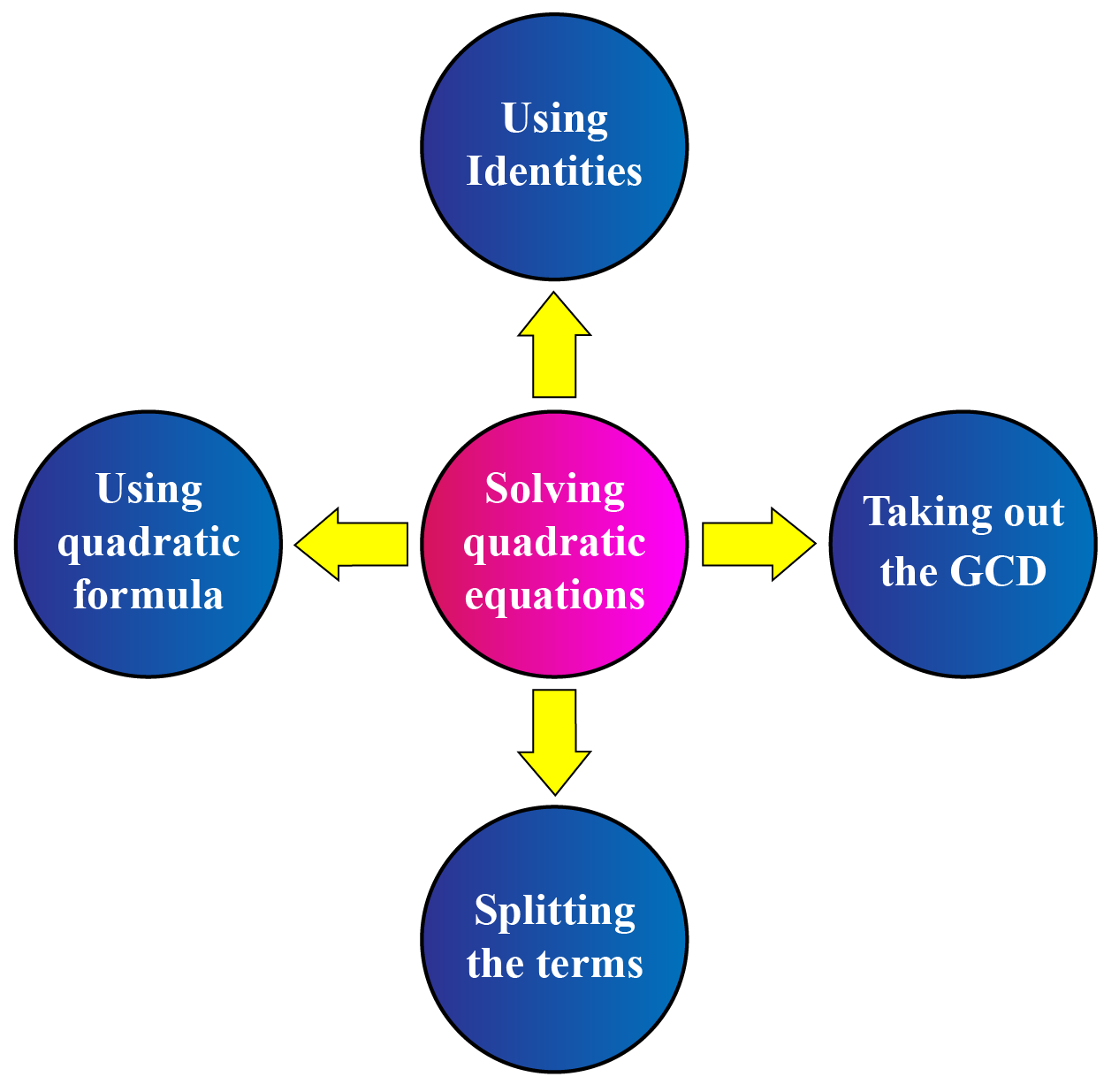### Find the common numeric factor and the algebraic factors shared by the terms in the quadratic equation and take them out.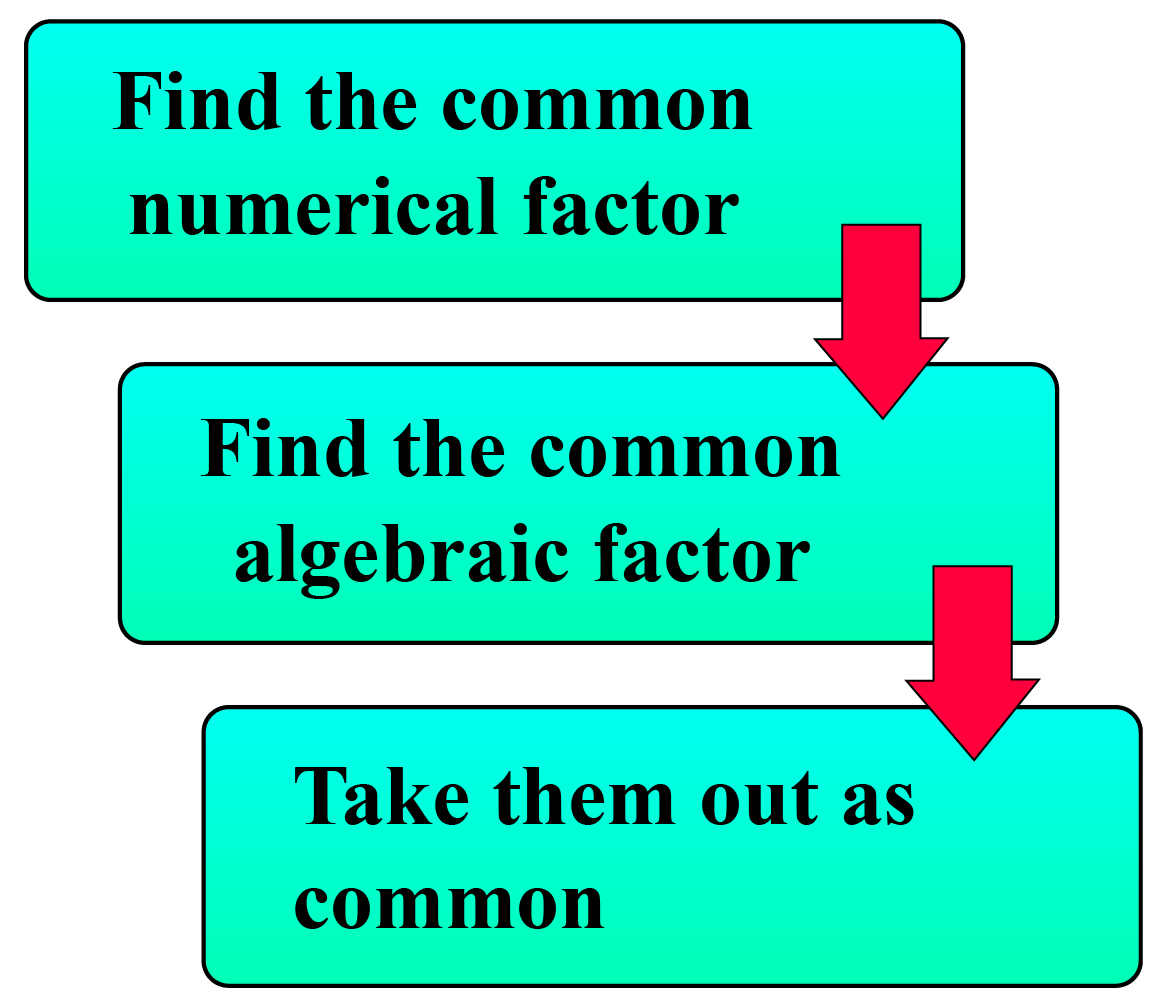Consider this example: $$3 x^2 + 6x = 0$$

• The numerical factor is 3 (coefficient of $$x^2$$) in both terms.
• The algebraic factor is x in both terms.
• The common factors are 3 and x. Hence take them out.
• Thus $$3 x^2 + 6x = 0$$ is factorized as $$3 x( x +2) = 0$$

### Splitting the middle terms

The sum of the roots in the quadratic equation $$\alpha + \beta = \dfrac{-b}{a}$$

The product of the roots in the quadratic equation $$\alpha\beta = \dfrac{c}{a}$$.

We split the middle term when we try to factorize to get the roots.

Factorize the third term in all possible ways and figure out the factors of that constant term.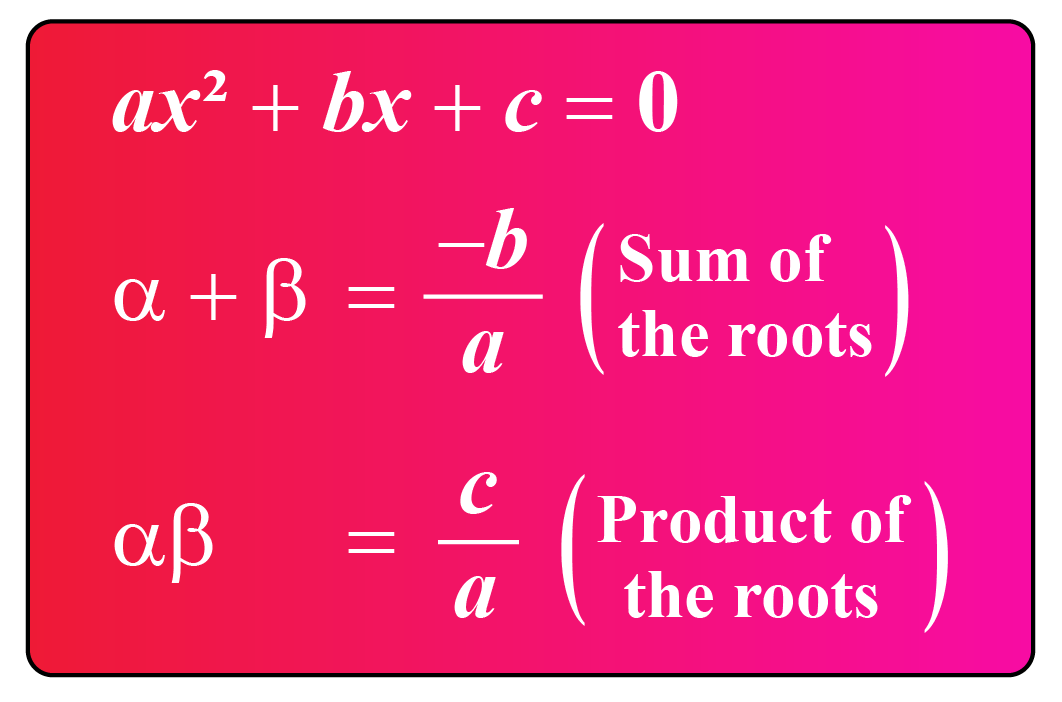Example: $$x2+8x+12$$

• We should split the middle term this way: $$x^2+ 8x + 12 = (x \pm \text{__}) (x \pm \text{__})$$
• The blanks should fit in this rule which should satisfy both the sum of the roots and the product of the roots.
• Here, the factors of 12 are $$1 \times12$$, $$2\times 6$$, $$3 \times 4$$
• Narrow down the possibilities this way.
Sum of the roots is 8 Product of the roots is 12
$$2+6 = 8$$ $$2\times 6 = 12$$
• Hence we choose 2 and 6 as the factors and group this way.

Grouping as per the table above,

$x^2+8x+12 = x ^ 2 + 2x + 6x +12$

Taking the common factors $$x$$ from the first two terms and 6 from the last two terms,

$x(x+2) + 6 (x+2)$

Taking the common factor (x+2) out to complete the problem

$(x+2) (x+6)$

Thus $$(x+2) (x+6)$$ are the factors of $$x^2+8x+12$$

### Using Algebraic Identities

Try to figure out if we could find out any algebraic identities that help us in getting the factorization done.

Example 1: $$9x^2 - 4$$

(difference of 2 perfect squares)

$9x^2 - 4 = (3x)^2 - 2^2$

We notice that this is of the form,

$a^2 - b ^2 = (a+b) (a-b)$

Hence we factorize the equation as $$(3x+2) (3x-2)$$

$9x^2 - 4 = (3x+2) (3x-2)$

Example 2: $$4x2+12x+9$$

$$4x2+12x+9 = (2x)^2 + 2(2x) (3) + (3)^2$$
We notice that this is of the form  $$(a+b)^2=(a^2 + 2ab + b^2)$$
Hence we have $$(2x+ 3) (2x+ 3)$$ as the linear factors.

Use this factorizing quadratic equation calculator and determine the roots and the factors.

If $$ax ^2 + bx +c = 0$$ is the quadratic equation,

$$a$$ is the coefficient of $$x ^2$$,

$$b$$ is the coefficient of $$x$$ and c is the constant.

Then we find the value of $$x$$ by using the formula:

 $$x= \dfrac{-b\pm \sqrt{b^2-4ac}}{2a}$$

Consider, $$x ^2 + 5x + 4 = 0$$

It is of the form $$ax ^2 + bx +c = 0$$

a is the coefficient of the first term, b is the coefficient of the middle term and c is the last term.

Here $$a =1$$, $$b = 5$$, $$c = 4$$

Substituting the values of a, b and c in this formula, we get

\begin{align}x&= \dfrac{-b\pm \sqrt{b^2-4ac}}{2a}\\\\x &= \dfrac{-5\pm \sqrt{5^2-4\times 1 \times 4}}{2\times 1}\\\\&= \dfrac{-5\pm \sqrt{25 -16}}{2}\\\\&= \dfrac{-5\pm \sqrt{9}}{2}\\\\&= \dfrac{-5\pm 3}{2}\end{align}

Here we get the 2 roots of the equation.

The two roots are found this way.

\begin{align}&= \dfrac{-5+3}{2}\text{ and } \dfrac{-5-3}{2}\\\\&=\dfrac{-2}{2}\text{ and }\dfrac{-8}{2}\\\\x &=(-1)\text{ and} (-4)\end{align}

$$\therefore$$ the factors are $$(x +1) (x+4)$$

## Solved Examples

 Example 1

Charles goes to the market to buy 2 packets of sugar and 1 packet of flour. The weight of the sugar is x and the weight of the flour is x times x. He can carry totally only 8 lbs in his bags. What could be the weight of each item?

SolutionLet us frame the equation.

$2 x + (1 x\times x) = 8$

$2 x + x^2 = 8$

This can be written in the standard form.

$x ^ 2 + 2 x - 8 = 0$

This is of the form of quadratic equation.

To find x, we need to factorize the quadratic.

\begin{align}x ^ 2 + 2 x - 8 &= 0\\x ^ 2 + 2x - 4x +8 &= 0\\ x (x + 2 ) - 4 ( x + 2) &= 0\\ (x -4)(x + 2) &= 0\end{align}

Thus Charles could buy 2 packs of 2 lbs sugar and 1 pack of 4 lb sugar.

 $$\therefore$$ Weight of the sugar = 2 lbs Weight of the flour = 4 lbs
 Example 2

Alex was teaching his students how to factorize $$2x^2 +9x +9 = 0$$ using the quadratic tiles and he arranged the tiles as follows. Can you verify if the factors of the equation are $$(x+3)$$ and $$(2x+3)$$?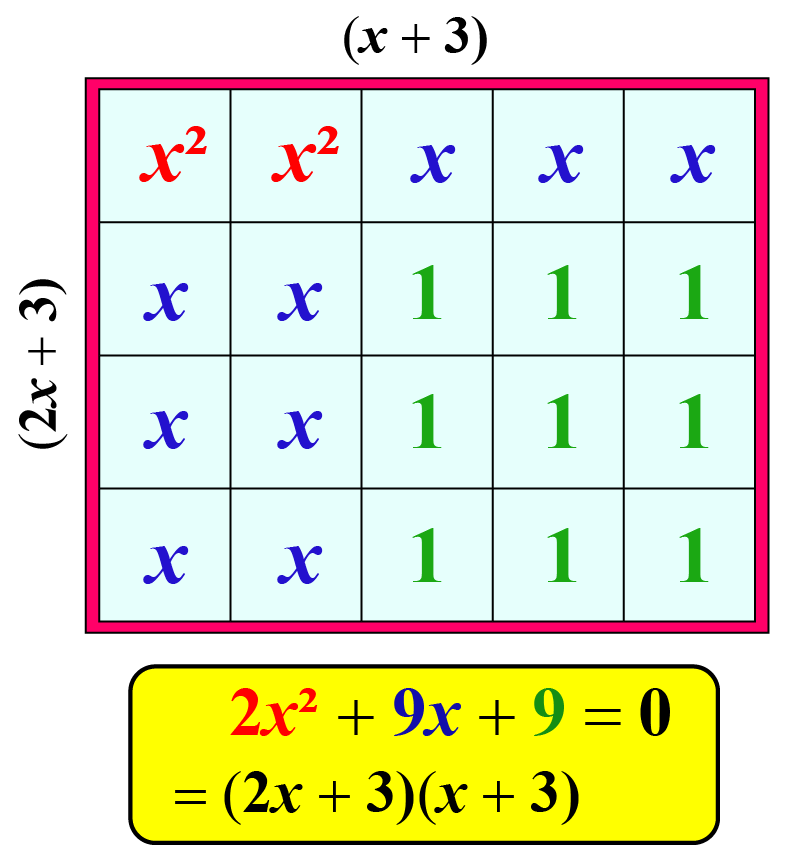Solution

To verify, we need to multiply the factors.

\begin{align}(2x+3) (x+3)\\ &= 2x ^2 +3 x +6x + 9\\ &= 2x ^2 + 9 x + 9\end{align}

Thus verified.

 $$\therefore 2x^2 +9x +9 = 0$$ is factored as $$(2x+3) (x+3)$$
 Example 3

Andrew is painting standing on the ladder. The ladder is 20 inches long. The difference between the height of the wall and the distance of the ladder from the wall is 4 inches. What could be their lengths?Solution

A right angle is formed between the wall and the distance of the ladder from the wall.

The length of the ladder is 20 inches.

Let the length of the sides be $$x$$ and $$x +4$$

Using Pythagorean theorem,

$x^2 + (x+4) ^2 = 20^2$

Expanding, $$(x+4)^2$$,

$x^2 + (x^2+16 + 8x) = 20^2$

Simplifying,

\begin{align}x^2 + x^2+16 + 8x &= 400\\\\2x^2+ 8x+16-400&=0\\\\2x^2 + 8x - 384 &=0\end{align}

Dividing by 2(coefficient of $$x^2$$) on both sides,

$x^2+4x-192 =0$

Factorizing,

$(x-12)(x+16)=0$

$x =12 \text{ or} x = -16$

Since the length cannot be in negative, we obtain $$x = 12$$

The other side would be $$x+4 = 12+4 = 16\text{ inches}$$

 $$\therefore$$The height of the wall = 16 in The distance of the ladder from the wall = 12 in
 Example 4

Shawn divides 25 in two parts such that the sum of their reciprocals is $$\dfrac{1}{6}$$. Can you find the parts?

Solution

Let x be one of the parts and the other part = 25 - x

The reciprocal of $$x = \dfrac{1}{x}$$

The reciprocal of $$25-x = \dfrac{1}{25-x}$$

The sum of reciprocals =   $$\dfrac{1}{x}+ \dfrac{1}{25-x} = \dfrac{1}{6}$$

Simplifying we get,

\begin{align}\dfrac{25- x+x}{x(25-x)} &= \dfrac{1}{6}\\\\ \dfrac{25}{x(25-x)} &= \dfrac{1}{6}\\\\ 25\times 16 &= x(25-x)\\\\ 150&= 25x -x^2\\\\ x^2 - 25x+150&= 0\end{align}

Let's use the method of quadratic formula to solve the equation.

Here $$a=1 , b= -25, c = 150$$

\begin{align}x&= \dfrac{-b\pm \sqrt{b^2-4ac}}{2a}\\\\x &= \dfrac{25\pm \sqrt{25^2-4\times 1 \times 150}}{2\times 1}\\\\&= \dfrac{25\pm \sqrt{625 -600}}{2}\\\\&= \dfrac{25\pm \sqrt{25}}{2}\\\\&= \dfrac{25\pm 5}{2}\end{align}
Thus the 2 roots are

\begin{align}&=\dfrac{25+5}{2} \text{and} \dfrac{25-5}{2}\\&=\dfrac{30}{2} \text{ and} \dfrac{20}{2}\\&=15, 10\end{align}

 $$\therefore$$Shawn divided 25 into two parts as 10 and 15.

## Interactive Questions

Here are a few activities for you to practice. Select/Type your answer and click the "Check Answer" button to see the result.Challenging Questions
• Find the measures of the rectangular garden whose area is is 256 $$units^2$$. Its width is 64 units less than the length of the garden.
• Solve: $$\dfrac{(2x-1)}{(x-1)} = \dfrac{(7x -1)}{(2x+2)}$$

## Let's Summarize

We hope you enjoyed learning about factorization of quadratic equations with interactive questions. Now, you will be able to easily solve problems on, quadratic equations formula and get the linear factors, by knowing the coeffiecients, by finding the sum and product of roots and knowing to use the factoring quadratic equation calculator.

At Cuemath, our team of math experts is dedicated to making learning fun for our favorite readers, the students!

Through an interactive and engaging learning-teaching-learning approach, the teachers explore all angles of a topic.

Be it worksheets, online classes, doubt sessions, or any other form of relation, it’s the logical thinking and smart learning approach that we, at Cuemath, believe in.

### 1. What is the formula to find the solution of a quadratic equation?

The formula to find the solution of a quadratic equation is $$x= \dfrac{-b\pm \sqrt{b^2-4ac}}{2a}$$, where a and b are the coefficients of $$x^2$$ and $$x$$ respectively and c is the constant term in the quadratic equation $$a x^2+ bx + c$$.

### 2. What are the methods to solve a quadratic equation?

The methods to solve a quadratic equation are splitting the terms, using algebraic identities, using the quadratic formula, and factoring the GCD out.

### 3. What is the method of factoring quadratic equations?

Splitting the terms and finding the sum and product of the roots is the best method of factoring quadratic equations.

### 4. Is factoring a quadratic equation the same as solving it?

Yes, we factor the quadratic equation and the next step is finding the zeros of the equation which is solving for the unknown variable.

### 5. What is the shortest way to factor any quadratic equation?

Using the quadratic formula is the shortest way to factor any quadratic equation.

### 6. Is using the quadratic formula easier than the factorization of the quadratic equation?

Using the quadratic formula is easier than factorization of a quadratic equation as it's easy to substitute the values of the coefficients in the formula.

### 7. What are some tricks for factorization of quadratic equations?

Find some of the roots and the products of the roots or by identifying any known algebraic identity, we can factorize the quadratic equations.

### 8. What is quadratic equation factored form?

$$(x - \alpha) (x - \beta)$$ is the factored form of quadratic equation.

### 10. How can you factorize easily?

By splitting the terms in such a way that the sum of the roots equal to the 2nd term's coefficient and the product of the roots equal to the value of the constant, we can do the factorization of the quadratic equations easily.

grade 10 | Questions Set 2